# SQL Query to Find Shortest & Longest String From a Column of a Table

• Difficulty Level : Basic
• Last Updated : 13 Apr, 2021

Here, we are going to see how to find the shortest and longest string from a column of a table in a database with the help of SQL queries. We will first create a database “geeks“, then we create a table “friends” with “firstName“, “lastName“, “age” columns. Then will perform our SQL query on this table to retrieve the shortest and longest string in a column.

For this article, we will be using the MS SQL Server as our database.

### Creating a Database :

Use the below SQL statement to create a database called geeks:

`CREATE DATABASE geeks;`

### Using Database :

`USE geeks;`

### Table Definition:

We have the following Employee table in our geeks database :

```CREATE TABLE friends(
firstName VARCHAR(30) not NULL,
lastName VARCHAR(30) not NULL,
age INT NOT NULL);```

You can use the below statement to query the description of the created table:

`EXEC SP_COLUMNS friends;`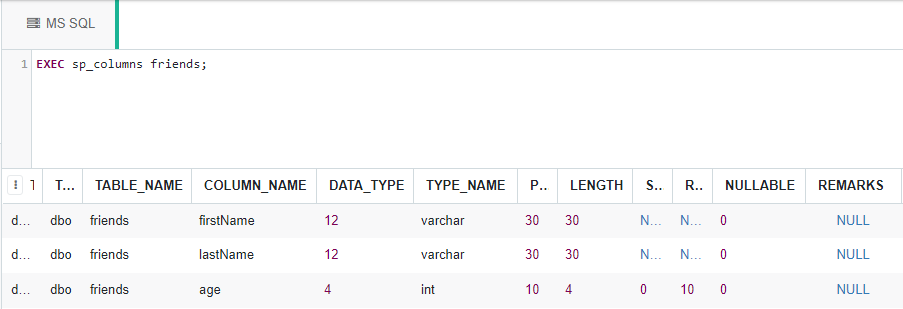### Adding Data to Table:

Use the below statement to add data to the friends table:

```INSERT INTO friends
values
('Ajay', 'More', 21),
('Zara', 'Khan', 20),
('Yogesh', 'Vaishnav', 21),
('Govind', 'Vaishnav', 22),
('Vishal', 'Vishwakarma', 21);```

To verify the contents of the table use the below statement:

`SELECT * FROM friends;`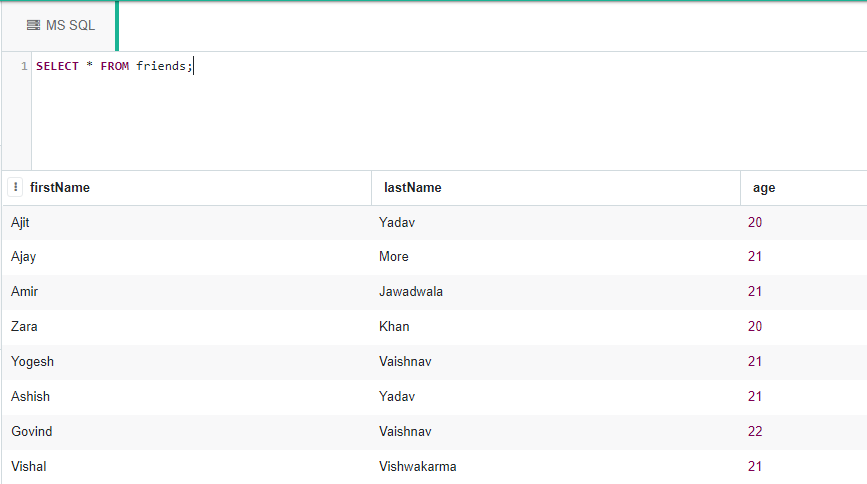Now let’s find the shortest and longest firstName from the table we have just created using char_length(), min(), and max() functions and LIMIT clause.

### The shortest firstName :

Use the ow syntax to find the shortest firstName in the friends table:

SYNTAX :

SELECT  TOP 1 *  FROM<table_name> –Here we want only one row that’s why TOP 1 *

WHERE

len(<string_column>) =

(SELECT min(len(<string_column>))  FROM<table_name> ) ;

Example :

```SELECT TOP 1 * FROM friends
WHERE
len(firstName) =
(SELECT min(len(firstName)) FROM friends);```

Output :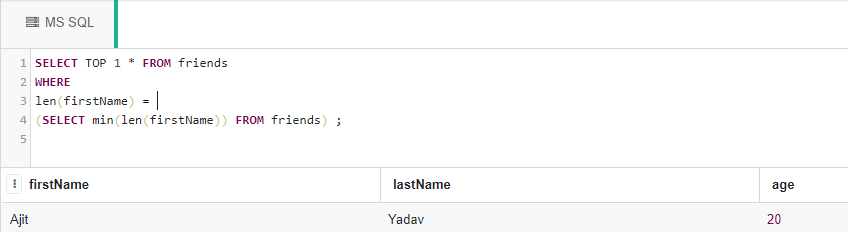### The row with lexicographically shortest firstName :

If there are more than one strings of the same minimum length, and we want to retrieve, lexicographically, the shortest string then can do as following:

SYNTAX :

SELECT TOP 1 *  FROM<table_name>  –Here we want only one row that’s why TOP 1*.

WHERE

len(<string_column>) =

(SELECTmin(len(<string_column>)) FROM <table_name> )

ORDER BY <column_name>;   –In this case column_name would be firstName.

Example :

```SELECT TOP 1 *  FROM friends
WHERE
len(firstName) =
(SELECT min(len(firstName)) FROM friends)
ORDER BY firstname;```

Output :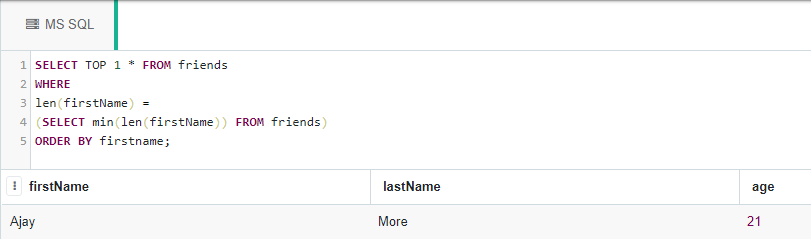### The row with longest firstName :

SYNTAX :

SELECT TOP 1* FROM<table_name>

WHERE

len(<string_column>) =

(SELECT max(len(<string_column>)) FROM <table_name> );

Example :

```SELECT TOP 1 * FROMfriends
WHERE
len(firstName) =
(SELECT max(len(firstName)) FROMfriends);```

Output :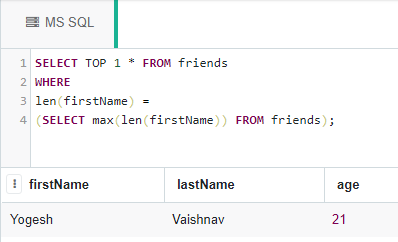### The row with lexicographically longest firstName :

SYNTAX :

SELECT TOP 1* FROM<table_name>

WHERE

len(<string_column>) =

(SELECT max(len(<string_column>)) from <table_name> )

ORDER BY <string_column>;  –here we would order data by firstName.

Example :

```SELECT TOP 1* FROM friends
WHERE
len(firstName) =
(SELECT max(len(firstName)) FROM friends)
ORDER BY firstName;```

Output :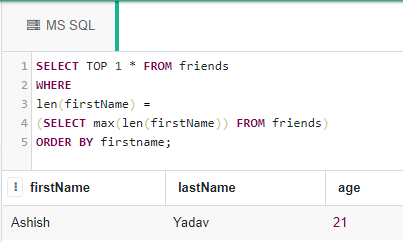My Personal Notes arrow_drop_up